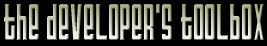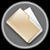This section of the archives stores flipcode's complete Developer Toolbox collection, featuring a variety of mini-articles and source code contributions from our readers.Projecting 3D Points   Submitted byIt is occasionally necessary to project points from 3D to 2D in software. A sample application is the rendering HUD effects like targeting boxes and character names in a game. The following code transforms and projects a 3D point or vector by the current OpenGL matrices and returns a 2D vector with associated depth value. The 2D vector is in the screen coordinate frame, where y increases from 0 at the top of the screen to height - 1 at the bottom and x increases from 0 at the left to width - 1 at the right. Morgan McGuire

 ```/*It is occasionally necessary to project points from 3D to 2D in software. A sample application is the rendering HUD effects like targeting boxes and character names in a game. The following code transforms and projects a 3D point or vector by the current OpenGL matrices and returns a 2D vector with associated depth value. The 2D vector is in the screen coordinate frame, where y increases from 0 at the top of the screen to height - 1 at the bottom and x increases from 0 at the left to width - 1 at the right.Morgan McGuire matrix@graphics3d.com*/Vector3 glProject(const Vector4& v) { // Get the matrices double modelView; double projection; glGetDoublev(GL_MODELVIEW_MATRIX, modelView); glGetDoublev(GL_PROJECTION_MATRIX, projection); // Compose the matrices into a net row-major transformation Vector4 transformation; for (int r = 0; r < 4; ++r) { for (int c = 0; c < 4; ++c) { transformation[r][c] = 0; for (int i = 0; i < 4; ++i) { // OpenGL matrices are column major transformation[r][c] += projection[r + i * 4] * modelView[i + c * 4]; } } } // Transform the vertex Vector4 result; for (r = 0; r < 4; ++r) { result[r] = transformation[r].dot(v); } // Homogeneous division const double rhw = 1 / result.w; return Vector3((result.x * rhw + 1) * width / 2, (1 - result.y * rhw) * height / 2, rhw); } ```